👈 Click

# Please open on desktop 🤷🏼‍♂️

## Line plot: number of earthquakes by year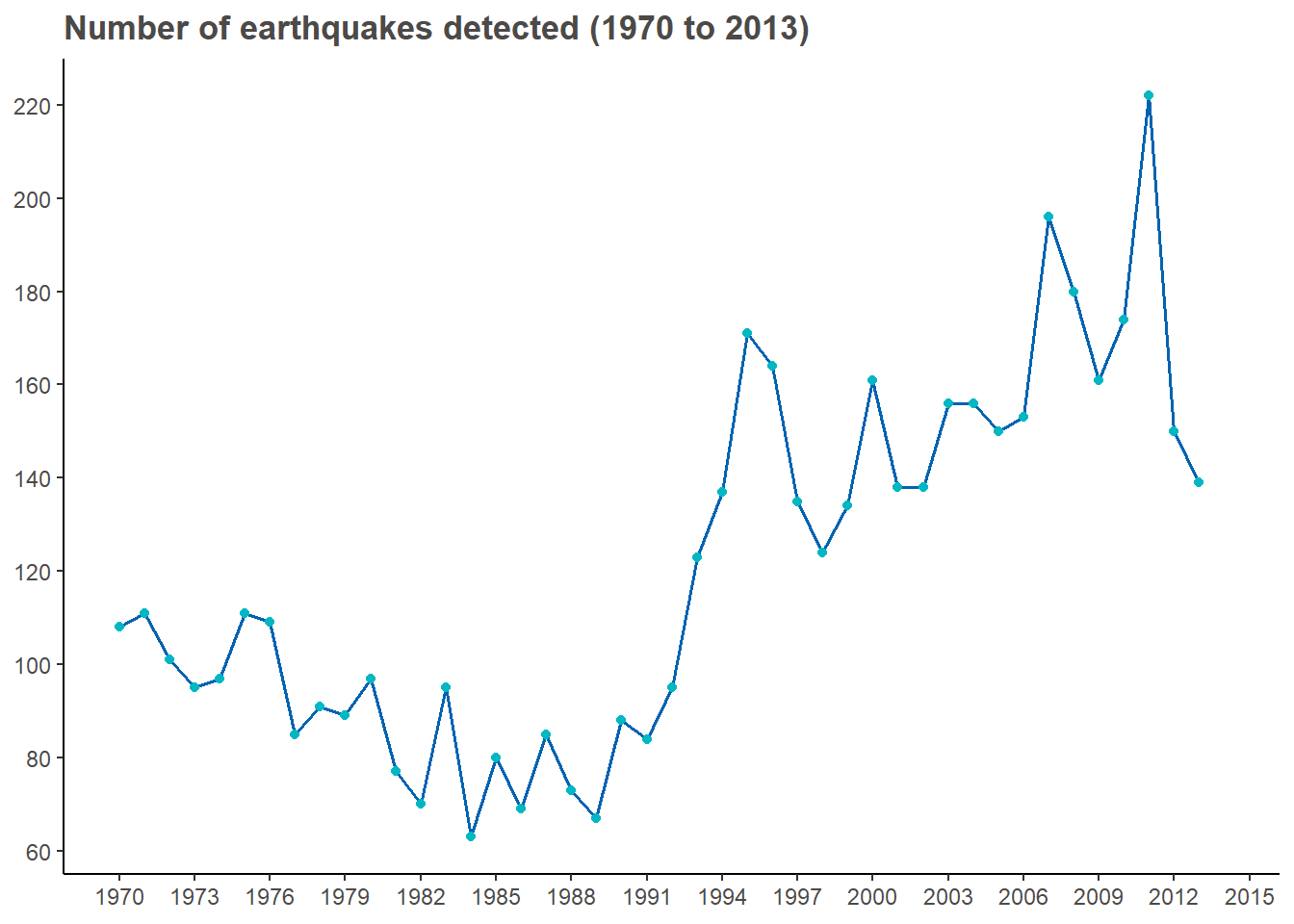The line chart suggests a general trend of increase in number of earthquakes detected. Notably, there was a significant increase in the number of earthquakes detected from 1991 to 1995. Is it true that we are experiencing a lot more seismicity than we used to? Not necessarily. The most reasonable interpretation of this line chart is that our ability to detect and measure earthquakes has improved over time with the technology advancement in the past few decades.

## Scatter plot: relationship between depth and magnitude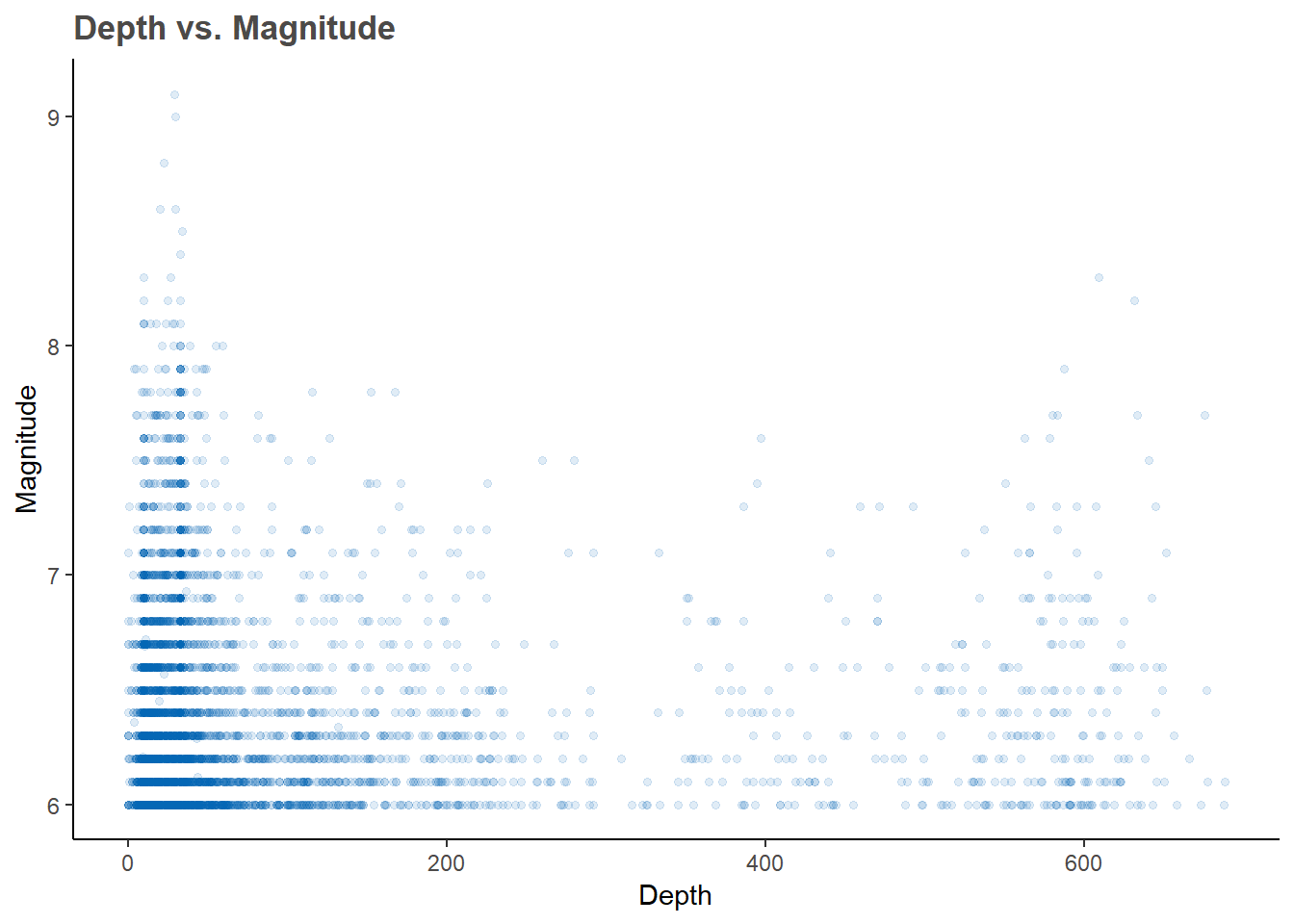The scatter plot suggests that there is no strong linear relationship between depth of the earthquake and its magnitude. The distribuiton is highly skewed to the left, indicating that the marjority of the earthquakes occured at a depth within 200km below the surface of the earth.

## Density plot: overall distribution of magnitudes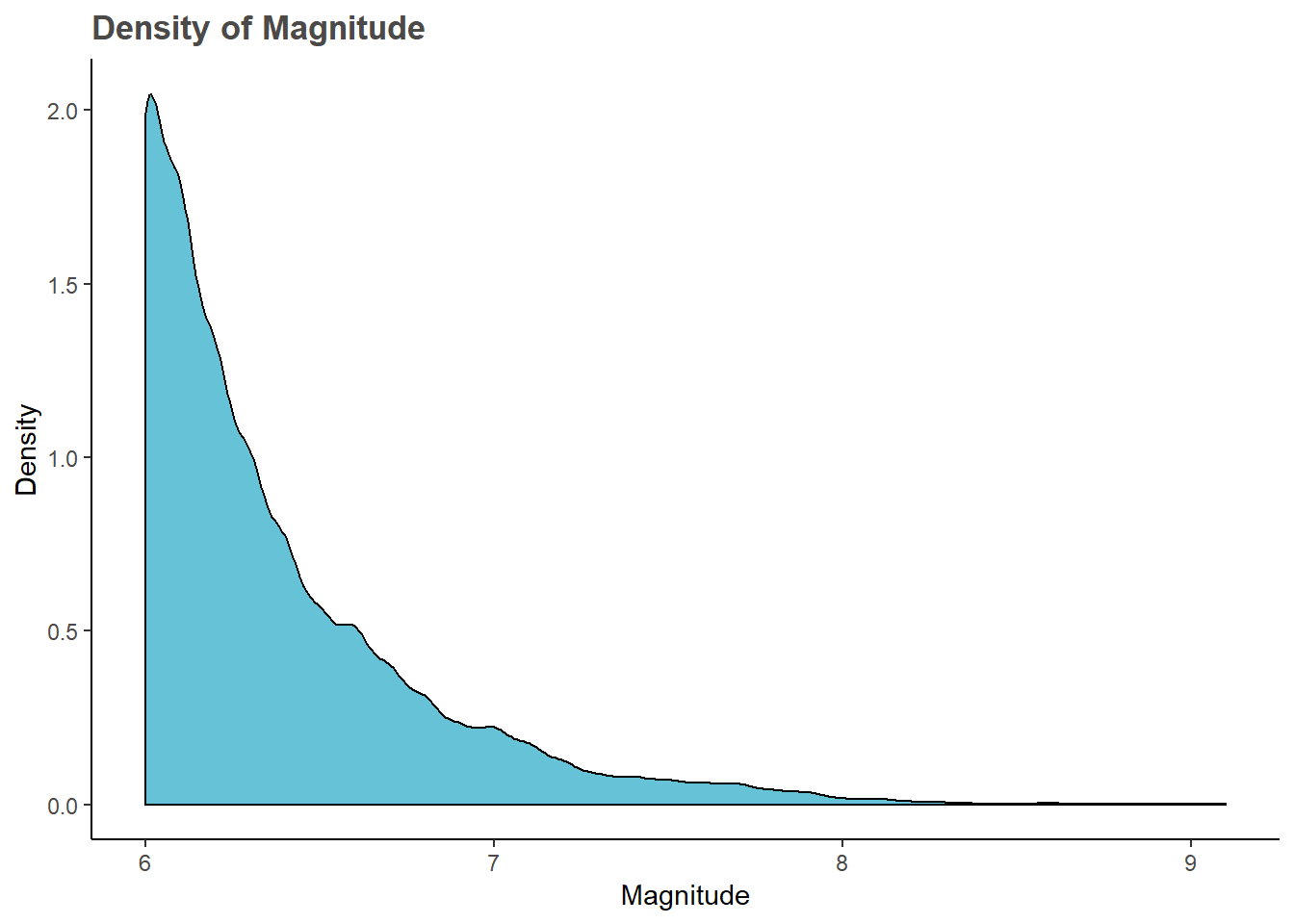The density plot shows the distribution of magnitudes of all earthquakes in the dataset. As shown in the graph, most earthquakes have a magnitude between 6 and 7.

## Density plot: distribution of magnitudes (top 10 countries by number of earthquakes detected)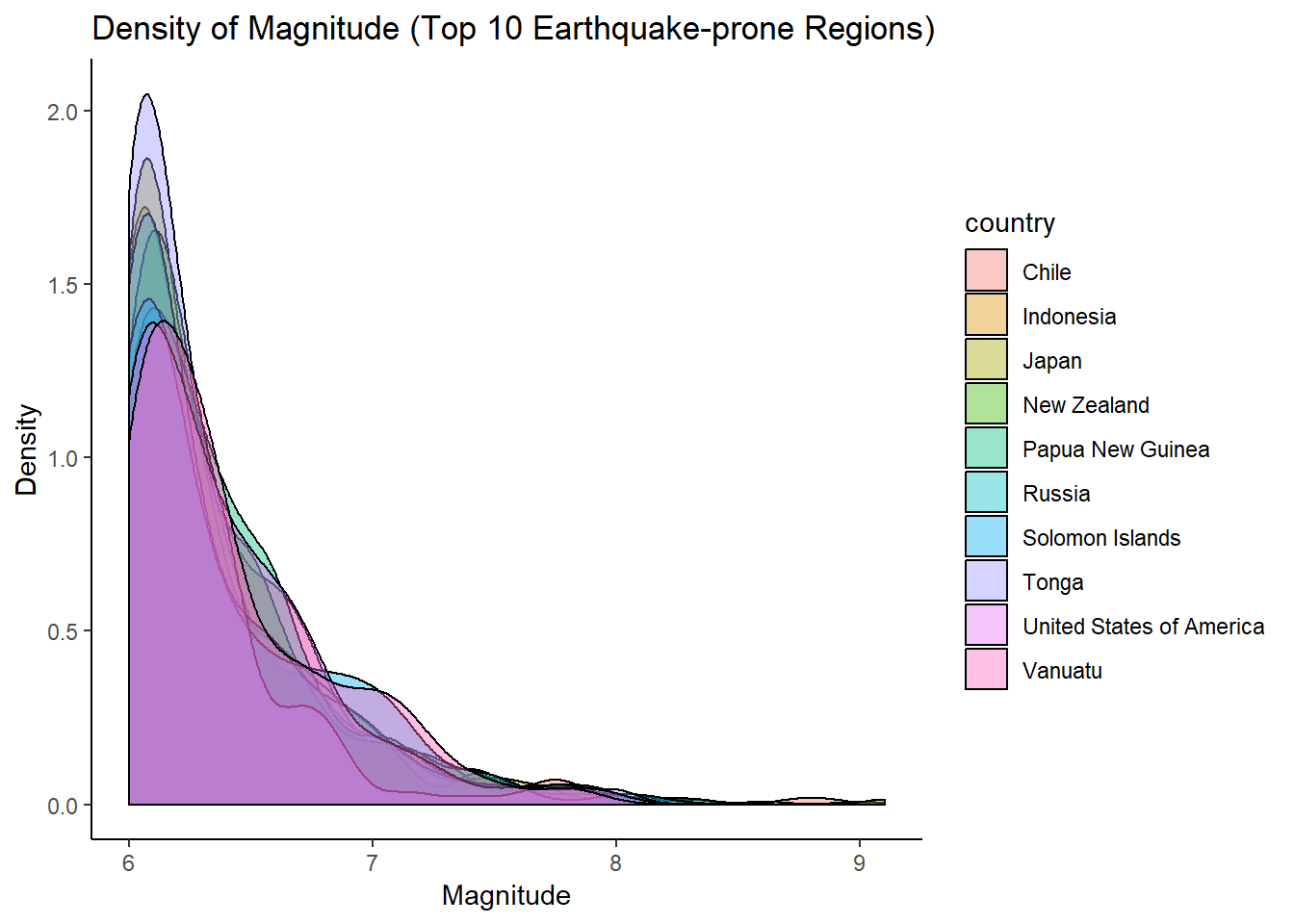In this density plot, we selected the top 10 earthquake-prone countries and plotted the distribution of magnitudes of the earthquakes detected in each country. Consistent with the previous chart, most earthquakes have a magnitude between 6 and 7, while earthquakes with magnitudes above 7 have slightly higher frequncies in the United States and Vanuatu.

## Bar chart: number of earthquakes (top 10 countries by number of earthquakes detected)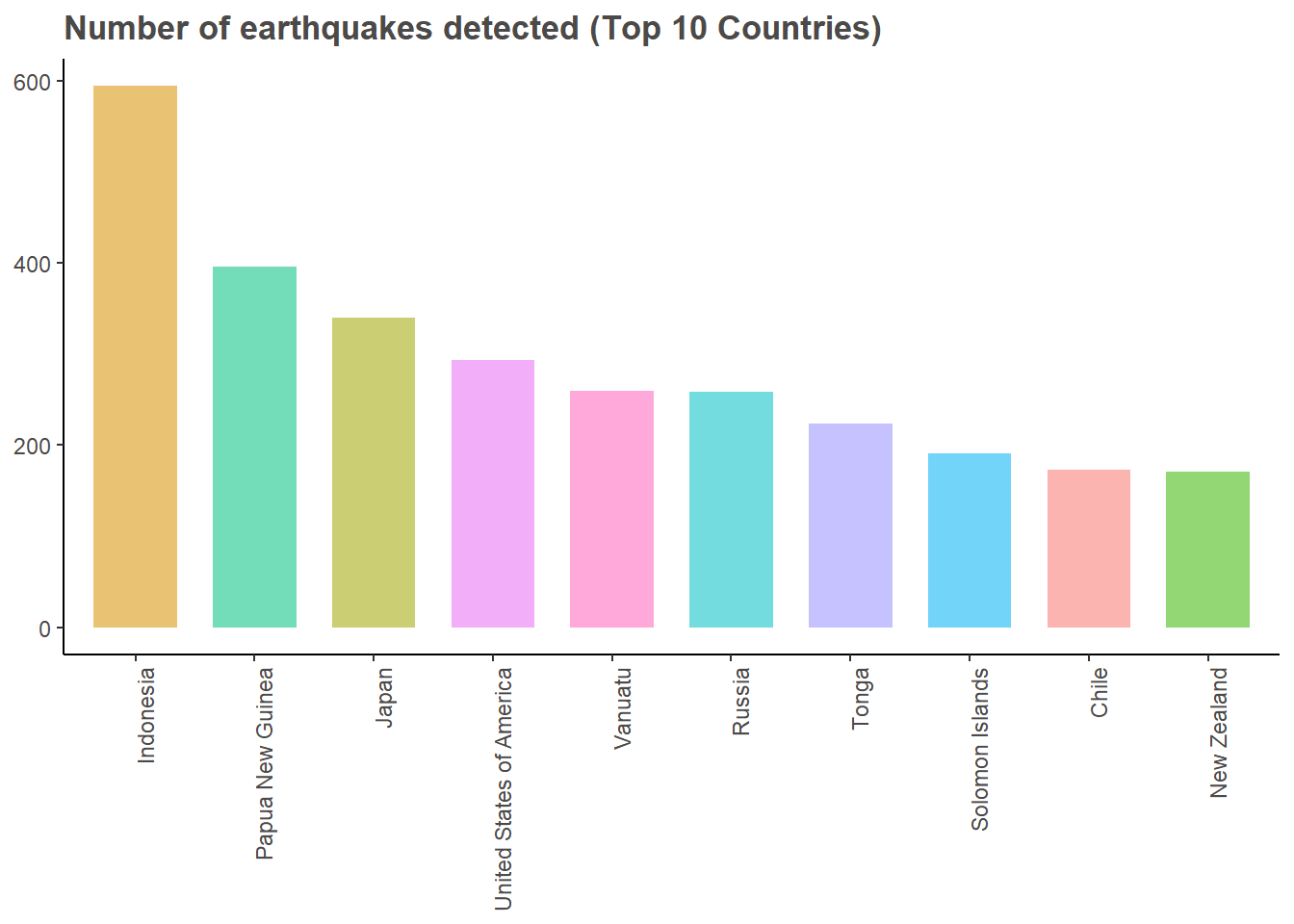According to this bar chart, Indonesia is the most earthquake-prone country in the world with a total number of 595 earthquakes (strong and above) detected from 1970 to 2013, followed by Papua New Guinea (396) and Japan (340).

## Box plot: magnitude (top 10 countries by number of earthquakes detected)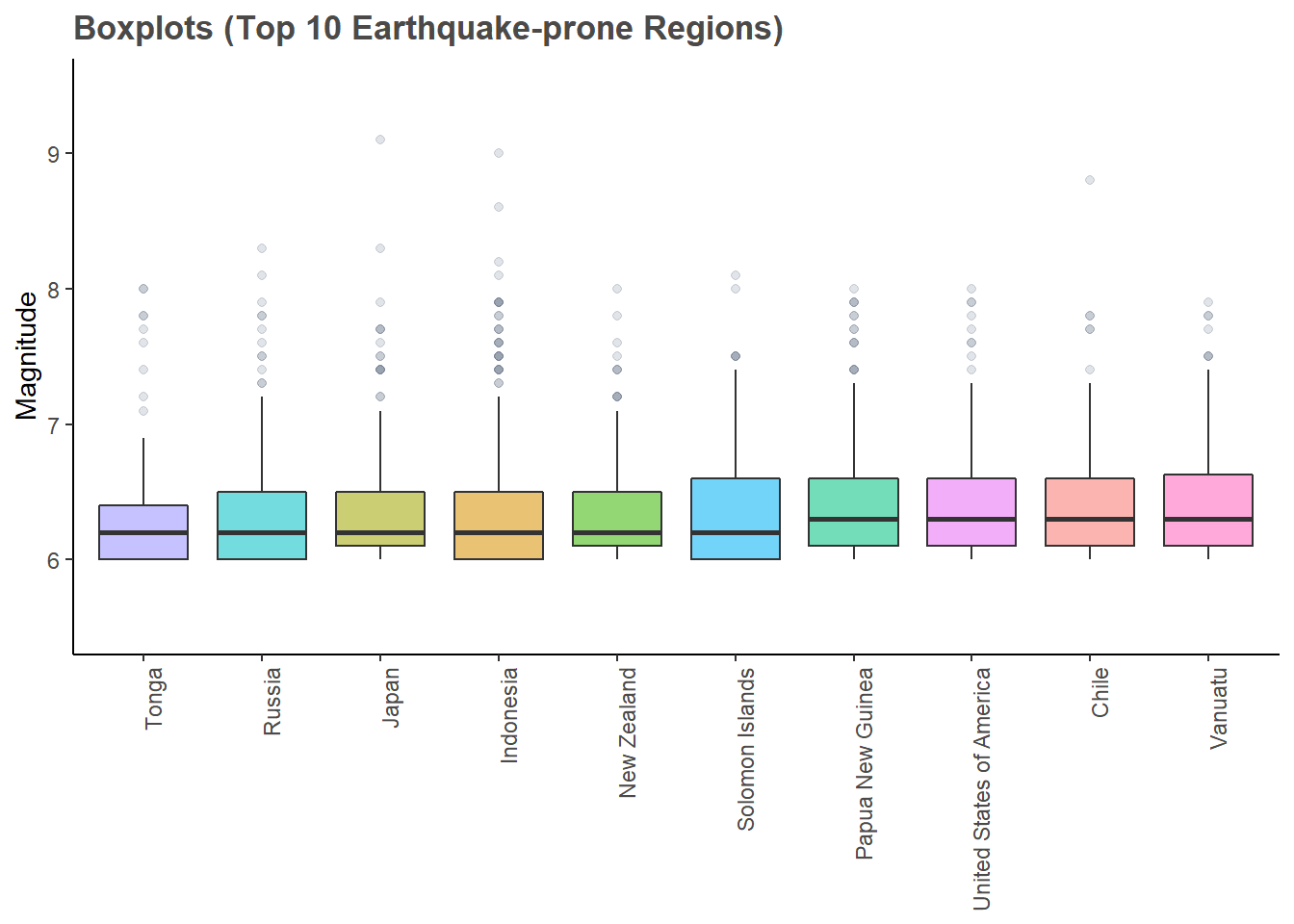The boxplots confirmed the observation from the desity plot that the distribution of magnitudes is highly skewed to the left (between 6 and 7). In addition, the boxplots reveals some interesting information regarding the outliers. According to the chart, Indonesia and Japans suffered the most from major (magnitude > 7) and great earthquakes (magnitude >8), suggeted by a high number of outliers outside the boxplot.

## Waffle chart: number of earthquakes by magnitude classes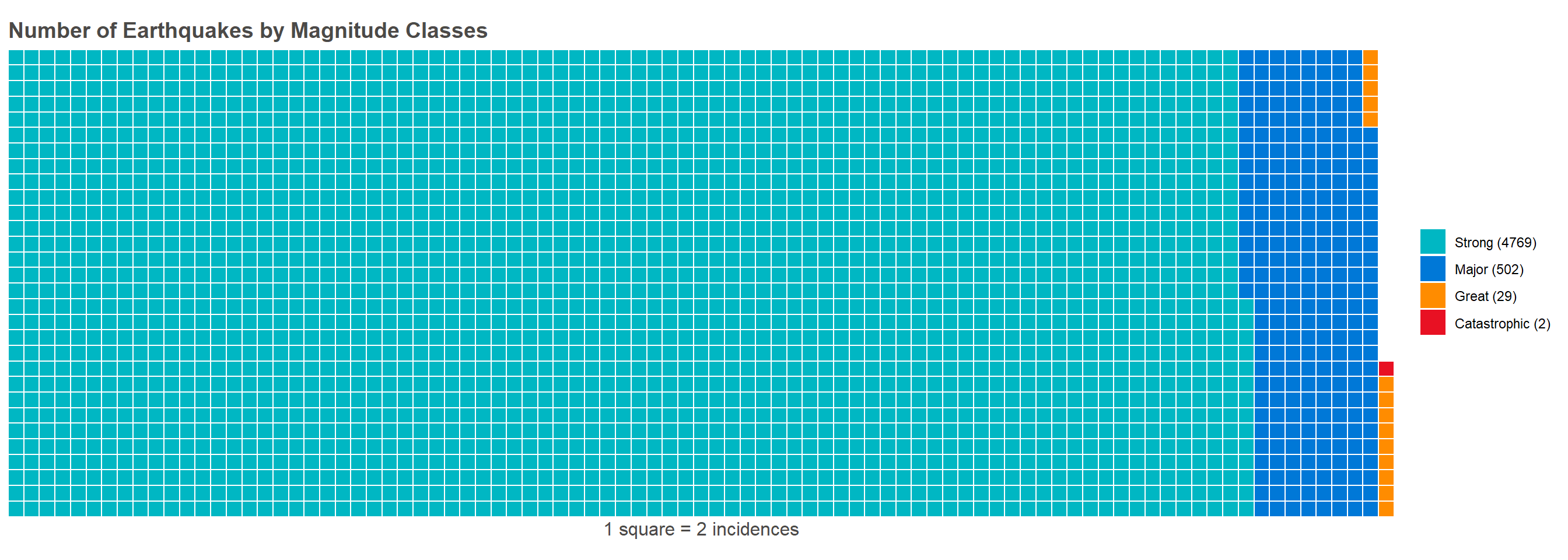The above waflle chart represents a more intuitive illustration of how the different classes of earthquakes are distributed. Consitent with what we have discussed earlier, marjority of the earthquakes have a magnitude between 6 and 7 (class ‘strong’). Earthquakes with magnitude above 8 have much fewer occurances, and two catastrophic earthquakes (magnitude above 9) occured in the past few decades.

## Waffle chart: number of earthquakes by countries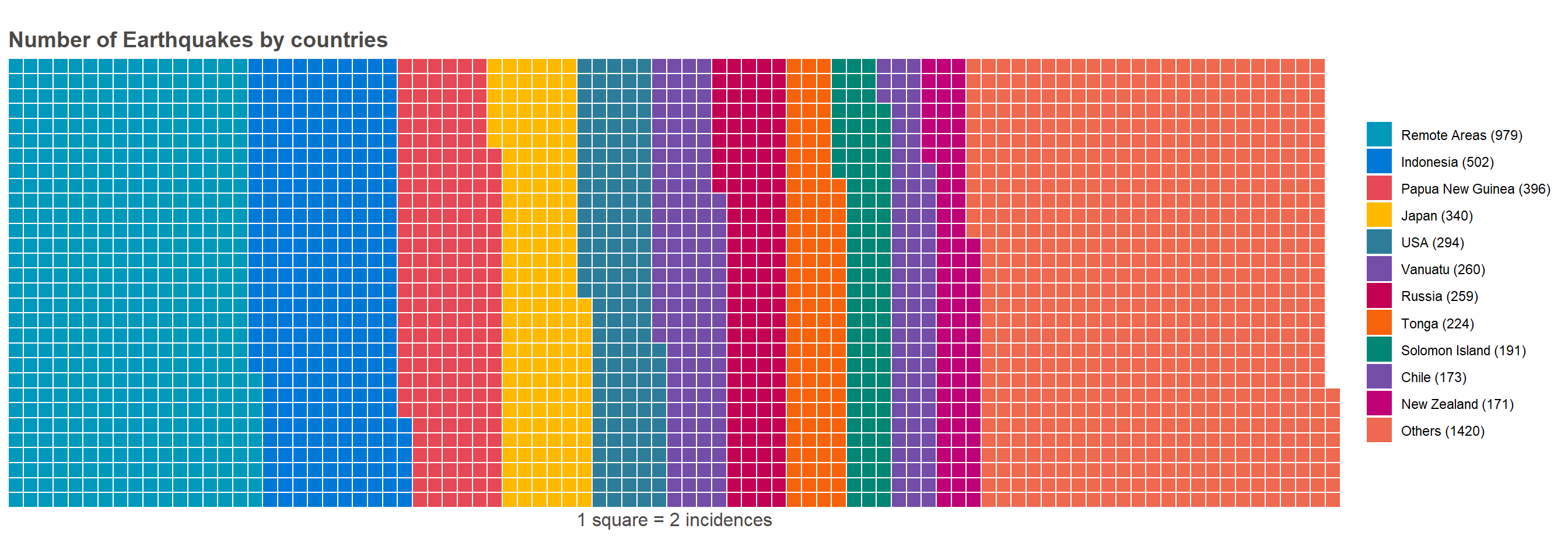This waffle chart visualizes how many earthquakes occured in each country (top 10). In addition to the top 10 earthquake-prone countries, earthquakes occured in remote areas (mostly in the oceans) are represented in this chart as well. As indicated by this chart, a high number of earhquakes (979) had occured in remote areas. More interestly, earthquakes in countries other than the top 10 were fewer than the top 10 combined.